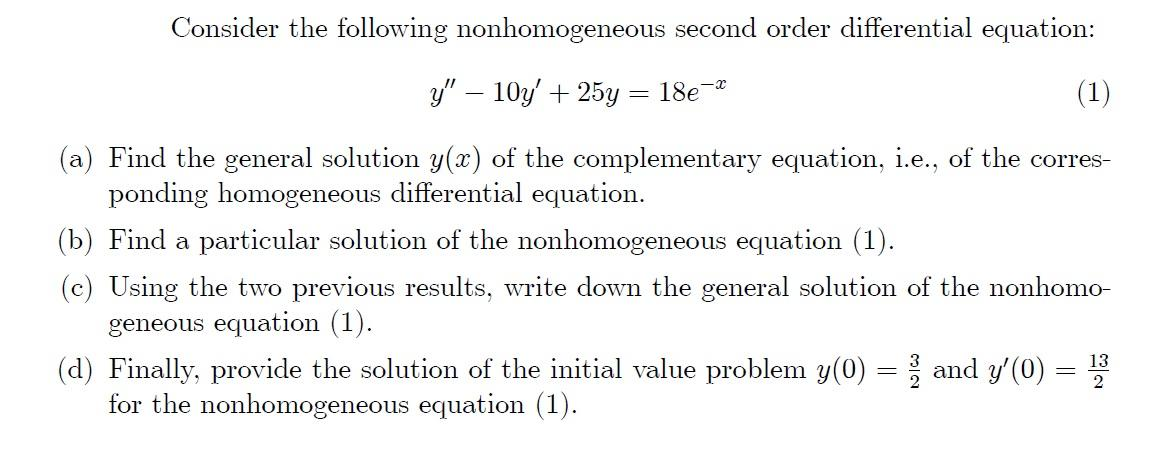### Create an Account

Already have account?

### Forgot Your Password ?

Home / Questions / Consider the following nonhomogeneous second order differential equation: y" – 10y' + 25y ...

# Consider the following nonhomogeneous second order differential equation: y" – 10y' + 25y = 18e (1) (a) Find the general solution y(x) of the complementary equation, i.e., of the corres- ponding homog

Consider the following nonhomogeneous second order differential equation: y" – 10y' + 25y = 18e (1) (a) Find the general solution y(x) of the complementary equation, i.e., of the corres- ponding homogeneous differential equation. (b) Find a particular solution of the nonhomogeneous equation (1). (c) Using the two previous results, write down the general solution of the nonhomo- geneous equation (1). (d) Finally, provide the solution of the initial value problem y(0) = 1 and y'(0) for the nonhomogeneous equation (1). ClaApr 14 2021 View more View Less

#### Answer (Solved)Subscribe To Get Solution